Have a question? Connect with the community at the TensorFlow Forum

# Data augmentation

## Overview

This tutorial demonstrates data augmentation: a technique to increase the diversity of your training set by applying random (but realistic) transformations such as image rotation. You will learn how to apply data augmentation in two ways. First, you will use Keras Preprocessing Layers. Next, you will use `tf.image`.

## Setup

``````import matplotlib.pyplot as plt
import numpy as np
import tensorflow as tf
import tensorflow_datasets as tfds

from tensorflow.keras import layers
``````

## Download a dataset

This tutorial uses the tf_flowers dataset. For convenience, download the dataset using TensorFlow Datasets. If you would like to learn about others ways of importing data, see the load images tutorial.

``````(train_ds, val_ds, test_ds), metadata = tfds.load(
'tf_flowers',
split=['train[:80%]', 'train[80%:90%]', 'train[90%:]'],
with_info=True,
as_supervised=True,
)
``````

The flowers dataset has five classes.

``````num_classes = metadata.features['label'].num_classes
print(num_classes)
``````
```5
```

Let's retrieve an image from the dataset and use it to demonstrate data augmentation.

``````get_label_name = metadata.features['label'].int2str

image, label = next(iter(train_ds))
_ = plt.imshow(image)
_ = plt.title(get_label_name(label))
``````## Use Keras preprocessing layers

### Resizing and rescaling

You can use preprocessing layers to resize your images to a consistent shape, and to rescale pixel values.

``````IMG_SIZE = 180

resize_and_rescale = tf.keras.Sequential([
layers.experimental.preprocessing.Resizing(IMG_SIZE, IMG_SIZE),
layers.experimental.preprocessing.Rescaling(1./255)
])
``````

You can see the result of applying these layers to an image.

``````result = resize_and_rescale(image)
_ = plt.imshow(result)
``````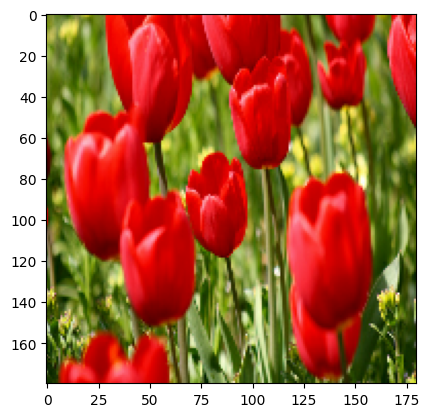You can verify the pixels are in `[0-1]`.

``````print("Min and max pixel values:", result.numpy().min(), result.numpy().max())
``````
```Min and max pixel values: 0.0 1.0
```

### Data augmentation

You can use preprocessing layers for data augmentation as well.

Let's create a few preprocessing layers and apply them repeatedly to the same image.

``````data_augmentation = tf.keras.Sequential([
layers.experimental.preprocessing.RandomFlip("horizontal_and_vertical"),
layers.experimental.preprocessing.RandomRotation(0.2),
])
``````
``````# Add the image to a batch
image = tf.expand_dims(image, 0)
``````
``````plt.figure(figsize=(10, 10))
for i in range(9):
augmented_image = data_augmentation(image)
ax = plt.subplot(3, 3, i + 1)
plt.imshow(augmented_image)
plt.axis("off")
``````There are a variety of preprocessing layers you can use for data augmentation including `layers.RandomContrast`, `layers.RandomCrop`, `layers.RandomZoom`, and others.

### Two options to use the preprocessing layers

There are two ways you can use these preprocessing layers, with important tradeoffs.

#### Option 1: Make the preprocessing layers part of your model

``````model = tf.keras.Sequential([
resize_and_rescale,
data_augmentation,
layers.Conv2D(16, 3, padding='same', activation='relu'),
layers.MaxPooling2D(),
# Rest of your model
])
``````

There are two important points to be aware of in this case:

• Data augmentation will run on-device, synchronously with the rest of your layers, and benefit from GPU acceleration.

• When you export your model using `model.save`, the preprocessing layers will be saved along with the rest of your model. If you later deploy this model, it will automatically standardize images (according to the configuration of your layers). This can save you from the effort of having to reimplement that logic server-side.

#### Option 2: Apply the preprocessing layers to your dataset

``````aug_ds = train_ds.map(
lambda x, y: (resize_and_rescale(x, training=True), y))
``````

With this approach, you use `Dataset.map` to create a dataset that yields batches of augmented images. In this case:

• Data augmentation will happen asynchronously on the CPU, and is non-blocking. You can overlap the training of your model on the GPU with data preprocessing, using `Dataset.prefetch`, shown below.
• In this case the prepreprocessing layers will not be exported with the model when you call `model.save`. You will need to attach them to your model before saving it or reimplement them server-side. After training, you can attach the preprocessing layers before export.

You can find an example of the first option in the image classification tutorial. Let's demonstrate the second option here.

### Apply the preprocessing layers to the datasets

Configure the train, validation, and test datasets with the preprocessing layers you created above. You will also configure the datasets for performance, using parallel reads and buffered prefetching to yield batches from disk without I/O become blocking. You can learn more dataset performance in the Better performance with the tf.data API guide.

``````batch_size = 32
AUTOTUNE = tf.data.AUTOTUNE

def prepare(ds, shuffle=False, augment=False):
# Resize and rescale all datasets
ds = ds.map(lambda x, y: (resize_and_rescale(x), y),
num_parallel_calls=AUTOTUNE)

if shuffle:
ds = ds.shuffle(1000)

# Batch all datasets
ds = ds.batch(batch_size)

# Use data augmentation only on the training set
if augment:
ds = ds.map(lambda x, y: (data_augmentation(x, training=True), y),
num_parallel_calls=AUTOTUNE)

# Use buffered prefecting on all datasets
return ds.prefetch(buffer_size=AUTOTUNE)
``````
``````train_ds = prepare(train_ds, shuffle=True, augment=True)
val_ds = prepare(val_ds)
test_ds = prepare(test_ds)
``````

### Train a model

For completeness, you will now train a model using these datasets. This model has not been tuned for accuracy (the goal is to show you the mechanics).

``````model = tf.keras.Sequential([
layers.Conv2D(16, 3, padding='same', activation='relu'),
layers.MaxPooling2D(),
layers.Conv2D(32, 3, padding='same', activation='relu'),
layers.MaxPooling2D(),
layers.Conv2D(64, 3, padding='same', activation='relu'),
layers.MaxPooling2D(),
layers.Flatten(),
layers.Dense(128, activation='relu'),
layers.Dense(num_classes)
])
``````
``````model.compile(optimizer='adam',
loss=tf.keras.losses.SparseCategoricalCrossentropy(from_logits=True),
metrics=['accuracy'])
``````
``````epochs=5
history = model.fit(
train_ds,
validation_data=val_ds,
epochs=epochs
)
``````
```Epoch 1/5
92/92 [==============================] - 14s 117ms/step - loss: 1.3954 - accuracy: 0.3934 - val_loss: 1.2173 - val_accuracy: 0.5177
Epoch 2/5
92/92 [==============================] - 3s 25ms/step - loss: 1.0951 - accuracy: 0.5555 - val_loss: 1.0964 - val_accuracy: 0.5668
Epoch 3/5
92/92 [==============================] - 3s 25ms/step - loss: 0.9855 - accuracy: 0.6032 - val_loss: 0.9367 - val_accuracy: 0.6349
Epoch 4/5
92/92 [==============================] - 3s 25ms/step - loss: 0.9228 - accuracy: 0.6308 - val_loss: 0.8939 - val_accuracy: 0.6594
Epoch 5/5
92/92 [==============================] - 3s 25ms/step - loss: 0.8850 - accuracy: 0.6536 - val_loss: 0.8712 - val_accuracy: 0.6512
```
``````loss, acc = model.evaluate(test_ds)
print("Accuracy", acc)
``````
```12/12 [==============================] - 4s 12ms/step - loss: 0.8603 - accuracy: 0.6458
Accuracy 0.6457765698432922
```

### Custom data augmentation

You can also create custom data augmenation layers. This tutorial shows two ways of doing so. First, you will create a `layers.Lambda` layer. This is a good way to write concise code. Next, you will write a new layer via subclassing, which gives you more control. Both layers will randomly invert the colors in an image, according to some probability.

``````def random_invert_img(x, p=0.5):
if  tf.random.uniform([]) < p:
x = (255-x)
else:
x
return x
``````
``````def random_invert(factor=0.5):
return layers.Lambda(lambda x: random_invert_img(x, factor))

random_invert = random_invert()
``````
``````plt.figure(figsize=(10, 10))
for i in range(9):
augmented_image = random_invert(image)
ax = plt.subplot(3, 3, i + 1)
plt.imshow(augmented_image.numpy().astype("uint8"))
plt.axis("off")
``````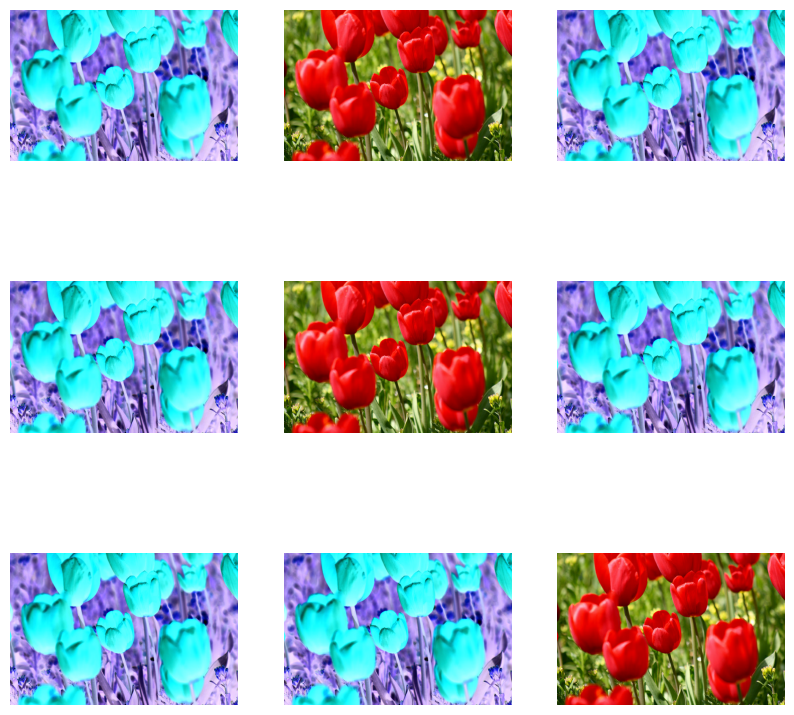Next, implement a custom layer by subclassing.

``````class RandomInvert(layers.Layer):
def __init__(self, factor=0.5, **kwargs):
super().__init__(**kwargs)
self.factor = factor

def call(self, x):
return random_invert_img(x)
``````
``````_ = plt.imshow(RandomInvert()(image))
``````Both of these layers can be used as described in options 1 and 2 above.

## Using tf.image

The above `layers.preprocessing` utilities are convenient. For finer control, you can write your own data augmentation pipelines or layers using `tf.data` and `tf.image`. You may also want to check out TensorFlow Addons Image: Operations and TensorFlow I/O: Color Space Conversions

Since the flowers dataset was previously configured with data augmentation, let's reimport it to start fresh.

``````(train_ds, val_ds, test_ds), metadata = tfds.load(
'tf_flowers',
split=['train[:80%]', 'train[80%:90%]', 'train[90%:]'],
with_info=True,
as_supervised=True,
)
``````

Retrieve an image to work with.

``````image, label = next(iter(train_ds))
_ = plt.imshow(image)
_ = plt.title(get_label_name(label))
``````Let's use the following function to visualize and compare the original and augmented images side-by-side.

``````def visualize(original, augmented):
fig = plt.figure()
plt.subplot(1,2,1)
plt.title('Original image')
plt.imshow(original)

plt.subplot(1,2,2)
plt.title('Augmented image')
plt.imshow(augmented)
``````

### Data augmentation

#### Flipping the image

Flip the image either vertically or horizontally.

``````flipped = tf.image.flip_left_right(image)
visualize(image, flipped)
``````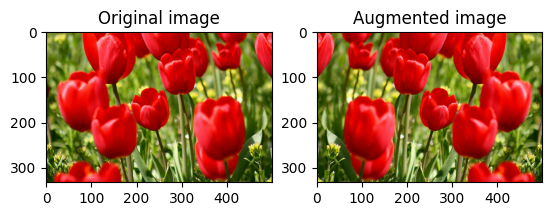#### Grayscale the image

Grayscale an image.

``````grayscaled = tf.image.rgb_to_grayscale(image)
visualize(image, tf.squeeze(grayscaled))
_ = plt.colorbar()
``````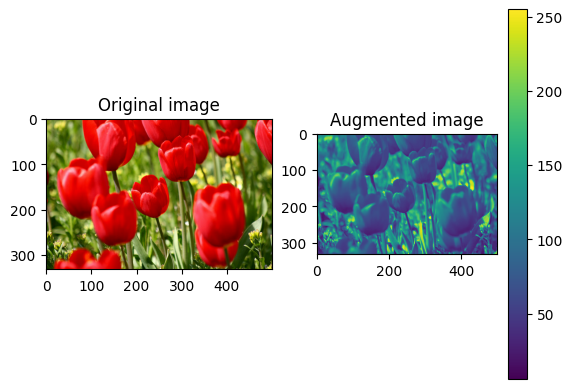#### Saturate the image

Saturate an image by providing a saturation factor.

``````saturated = tf.image.adjust_saturation(image, 3)
visualize(image, saturated)
``````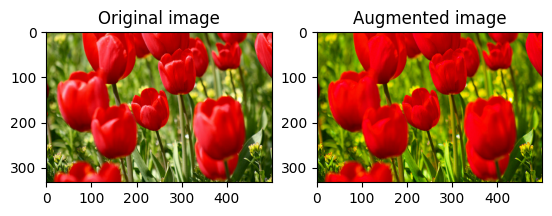#### Change image brightness

Change the brightness of image by providing a brightness factor.

``````bright = tf.image.adjust_brightness(image, 0.4)
visualize(image, bright)
``````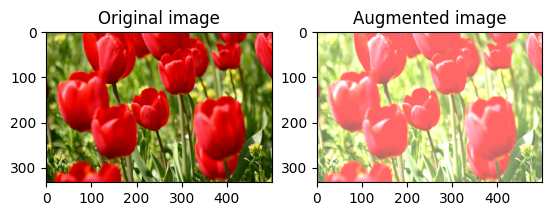#### Center crop the image

Crop the image from center up to the image part you desire.

``````cropped = tf.image.central_crop(image, central_fraction=0.5)
visualize(image,cropped)
``````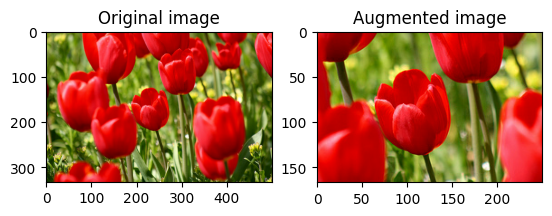#### Rotate the image

Rotate an image by 90 degrees.

``````rotated = tf.image.rot90(image)
visualize(image, rotated)
``````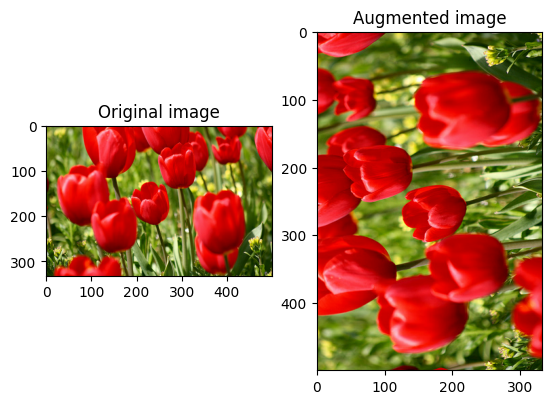### Random transformations

Applying random transformations to the images can further help generalize and expand the dataset. Current `tf.image` API provides 8 such random image operations (ops):

These random image ops are purely functional: the ouput only depends on the input. This makes them simple to use in high performance, deterministic input pipelines. They require a `seed` value be input each step. Given the same `seed`, they return the same results independent of how many times they are called.

In the following sections, we will:

1. Go over examples of using random image operations to transform an image, and
2. Demonstrate how to apply random transformations to a training dataset.

#### Randomly change image brightness

Randomly change the brightness of `image` by providing a brightness factor and `seed`. The brightness factor is chosen randomly in the range `[-max_delta, max_delta)` and is associated with the given `seed`.

``````for i in range(3):
seed = (i, 0)  # tuple of size (2,)
stateless_random_brightness = tf.image.stateless_random_brightness(
image, max_delta=0.95, seed=seed)
visualize(image, stateless_random_brightness)
``````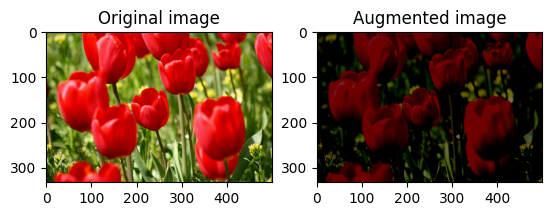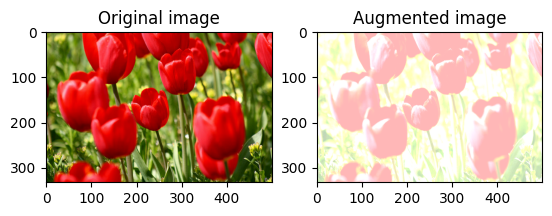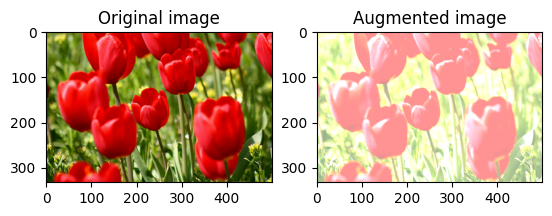#### Randomly change image contrast

Randomly change the contrast of `image` by providing a contrast range and `seed`. The contrast range is chosen randomly in the interval `[lower, upper]` and is associated with the given `seed`.

``````for i in range(3):
seed = (i, 0)  # tuple of size (2,)
stateless_random_contrast = tf.image.stateless_random_contrast(
image, lower=0.1, upper=0.9, seed=seed)
visualize(image, stateless_random_contrast)
``````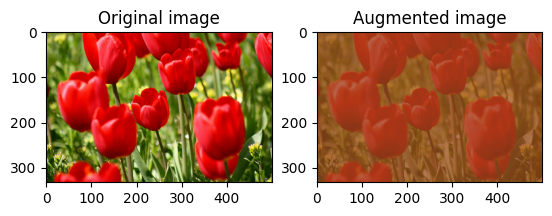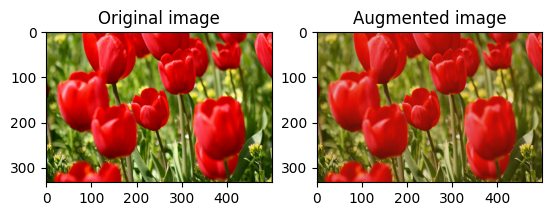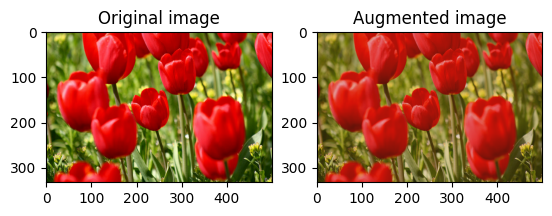#### Randomly crop an image

Randomly crop `image` by providing target `size` and `seed`. The portion that gets cropped out of `image` is at a randomly chosen offet and is associated with the given `seed`.

``````for i in range(3):
seed = (i, 0)  # tuple of size (2,)
stateless_random_crop = tf.image.stateless_random_crop(
image, size=[210, 300, 3], seed=seed)
visualize(image, stateless_random_crop)
``````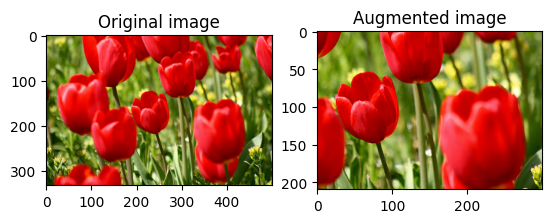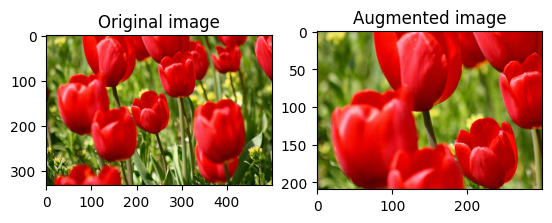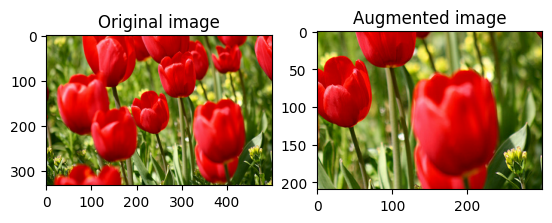### Apply augmentation to a dataset

Let's first download the image dataset again in case they are modified in the previous sections.

``````(train_datasets, val_ds, test_ds), metadata = tfds.load(
'tf_flowers',
split=['train[:80%]', 'train[80%:90%]', 'train[90%:]'],
with_info=True,
as_supervised=True,
)
``````

Let's define a utility function for resizing and rescaling the images. This function will be used in unifying the size and scale of images in the dataset:

``````def resize_and_rescale(image, label):
image = tf.cast(image, tf.float32)
image = tf.image.resize(image, [IMG_SIZE, IMG_SIZE])
image = (image / 255.0)
return image, label
``````

Let's also define `augment` function that can apply the random transformations to the images. This function will be used on the dataset in the next step.

``````def augment(image_label, seed):
image, label = image_label
image, label = resize_and_rescale(image, label)
image = tf.image.resize_with_crop_or_pad(image, IMG_SIZE + 6, IMG_SIZE + 6)
# Make a new seed
new_seed = tf.random.experimental.stateless_split(seed, num=1)[0, :]
# Random crop back to the original size
image = tf.image.stateless_random_crop(
image, size=[IMG_SIZE, IMG_SIZE, 3], seed=seed)
# Random brightness
image = tf.image.stateless_random_brightness(
image, max_delta=0.5, seed=new_seed)
image = tf.clip_by_value(image, 0, 1)
return image, label
``````

#### Option 1: Using `tf.data.experimental.Counter()`

Create a `tf.data.experimental.Counter()` object (let's call it `counter`) and `zip` the dataset with `(counter, counter)`. This will ensure that each image in the dataset gets associated with a unique value (of shape `(2,)`) based on `counter` which later can get passed into the `augment` function as the `seed` value for random transformations.

``````# Create counter and zip together with train dataset
counter = tf.data.experimental.Counter()
train_ds = tf.data.Dataset.zip((train_datasets, (counter, counter)))
``````

Map the `augment` function to the training dataset.

``````train_ds = (
train_ds
.shuffle(1000)
.map(augment, num_parallel_calls=AUTOTUNE)
.batch(batch_size)
.prefetch(AUTOTUNE)
)
``````
``````val_ds = (
val_ds
.map(resize_and_rescale, num_parallel_calls=AUTOTUNE)
.batch(batch_size)
.prefetch(AUTOTUNE)
)
``````
``````test_ds = (
test_ds
.map(resize_and_rescale, num_parallel_calls=AUTOTUNE)
.batch(batch_size)
.prefetch(AUTOTUNE)
)
``````

#### Option 2: Using `tf.random.Generator`

Create a `tf.random.Generator` object with an intial `seed` value. Calling `make_seeds` function on the same generator object returns a new, unique `seed` value always. Define a wrapper function that 1) calls `make_seeds` function and that 2) passes the newly generated `seed` value into the `augment` function for random transformations.

``````# Create a generator
rng = tf.random.Generator.from_seed(123, alg='philox')
``````
``````# A wrapper function for updating seeds
def f(x, y):
seed = rng.make_seeds(2)
image, label = augment((x, y), seed)
return image, label
``````

Map the wrapper function `f` to the training dataset.

``````train_ds = (
train_datasets
.shuffle(1000)
.map(f, num_parallel_calls=AUTOTUNE)
.batch(batch_size)
.prefetch(AUTOTUNE)
)
``````
``````val_ds = (
val_ds
.map(resize_and_rescale, num_parallel_calls=AUTOTUNE)
.batch(batch_size)
.prefetch(AUTOTUNE)
)
``````
``````test_ds = (
test_ds
.map(resize_and_rescale, num_parallel_calls=AUTOTUNE)
.batch(batch_size)
.prefetch(AUTOTUNE)
)
``````

These datasets can now be used to train a model as shown previously.

## Next steps

This tutorial demonstrated data augmentation using Keras Preprocessing Layers and `tf.image`. To learn how to include preprocessing layers inside your model, see the Image classification tutorial. You may also be interested in learning how preprocessing layers can help you classify text, as shown in the Basic text classification tutorial. You can learn more about `tf.data` in this guide, and you can learn how to configure your input pipelines for performance here.

[{ "type": "thumb-down", "id": "missingTheInformationINeed", "label":"Missing the information I need" },{ "type": "thumb-down", "id": "tooComplicatedTooManySteps", "label":"Too complicated / too many steps" },{ "type": "thumb-down", "id": "outOfDate", "label":"Out of date" },{ "type": "thumb-down", "id": "samplesCodeIssue", "label":"Samples / code issue" },{ "type": "thumb-down", "id": "otherDown", "label":"Other" }]
[{ "type": "thumb-up", "id": "easyToUnderstand", "label":"Easy to understand" },{ "type": "thumb-up", "id": "solvedMyProblem", "label":"Solved my problem" },{ "type": "thumb-up", "id": "otherUp", "label":"Other" }]